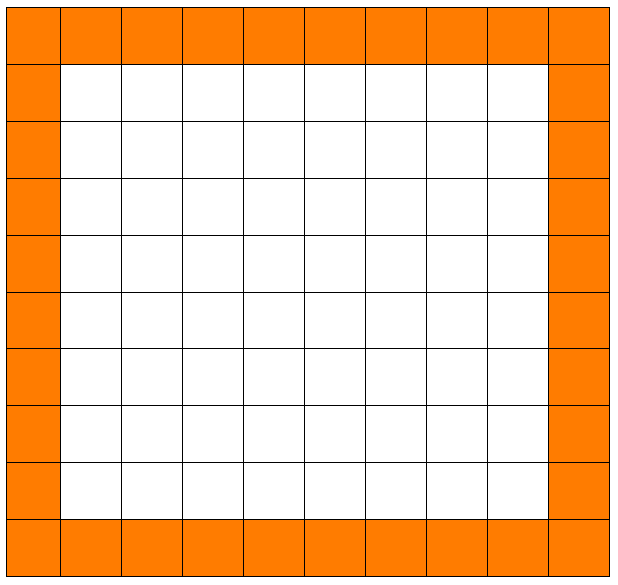Categories

# The Border Problem and Variables

In this 7th grade class, they’re studying interesting ways of counting up the boxes in the border of a square grid.They originally start with a 10 by 10 grid, but soon after they expand to a  6 by 6 gride and a 15 by 15 grid and use their techniques to count the squares in the new border. Then, in class, they introduce the notion of variable and set about shortening one of their verbal explanations using variables.

On the board are two expressions: 6 + 6 + 4 + 4 and 15 + 15 + 13 + 13. The teacher tasks the students with writing an algebraic expression that represents the number of squares on the border. The following is a recorded interaction among students during their group work:

Sharmeen: s + s + (s-2) + (s-2). Though that’s kind of complicated. Is there any other way to put it?

Antony: What is it?

Sharmeen: Uh, mine? It was s + s + (s-2) + (s-2)

Kim: No we had to, like, um, how about we write a variable for…make a variable for thirteen.

Sharmeen: Yeah, oops. Oh, m equals… OK, so it’s s + s + m + m.

What make you of this?

[All of this is lifted from “Connecting Mathematical Ideas“.]

## 3 replies on “The Border Problem and Variables”

Kim is making a mistake I’ve noticed a lot of my struggling students make, where they know that a variable can represent “anything,” but take this to mean that it should equal *something* in particular. I think this misunderstanding is furthered by (almost) always asking students to “solve for x.” In so doing, a lot of our students come to view the variable as a specific number that is in hiding, and our job is to uncover its value.

When it comes time to write our own expressions, this understanding of the variable hurts us, because it hides the fact that the variable represents a number that we can freely change–it doesn’t actually get “locked in” to a value until we set the expression to equal something.

If I were listening in on Sharmeen’s last comment, I might ask what “m” stands for in this case. Then what it would have to be in another case. Then if there was another way to express this just in terms of the side length. Hopefully we’d work our way back to where he started. I’d want to focus on the fact that the power of the variable comes from being able to determine what to plug in from the original problem statement. We can do that for the “s” variable, but once we introduce this “m” we can’t.

a lot of our students come to view the variable as a specific number that is in hiding

Good way of putting it. To me, it’s almost the difference between a variable representing some number, and a variable representing any number.

Iansays:

There is an example:
“Suppose that it takes Tom and Dick 2 hours to do a certain job, it takes Tom and Harry 3 hours to do the same job and it takes Dick and Harry 4 hours to do the same job. How long would it take Tom, Dick and Harry to do the same job if all 3 men worked together?”

A common way for pupils to represent this using algebra is as follows:

T + D = 2
T + H = 3
D + H = 4;
then they solve these equations for T,D and H and add-up the numbers
T + D + H = 4.5 hours.

Which is obviously the wrong answer as more men equals less time. But the fundamental problem is what do these letters represent. I think this is an area that a lot of students do not get. They groan when algebra is heard and most of the time one of the first things I hear when I start teaching a class is, “but what IS x?”

I think Chris makes an excellent point that these letters can mean anything we need them to be. For example we could easily have written the original problem as s-100 + s-100 + s-102 + s-102 and it is still the same thing. IT’s helping pupils to understand what the variable means.

The solution and argument for the Tom, Dick, Harry problem can be found here, in this paper:

https://www.dropbox.com/s/un82mmz8iyssyy6/algebraic%20word%20problems.pdf